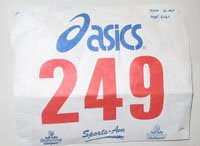## Monday, December 8, 2008

### 249

249 = 3 x 83.

The sum of the digits of 249 equals five times the number of digits: 2 + 4 + 9 = 15 = 3 x 5.

249 is a number not divisible by any of its digits.

249 is a Blum number. A natural number n is a Blum integer if n = pq is a semiprime (the product of two prime numbers) for which p and q are distinct prime numbers congruent to 3 mod 4. That is, p and q must be of the form 4t + 3, for some integer t.249 Ilse is an asteroid with an unusually slow rotation period, discovered in 1885.